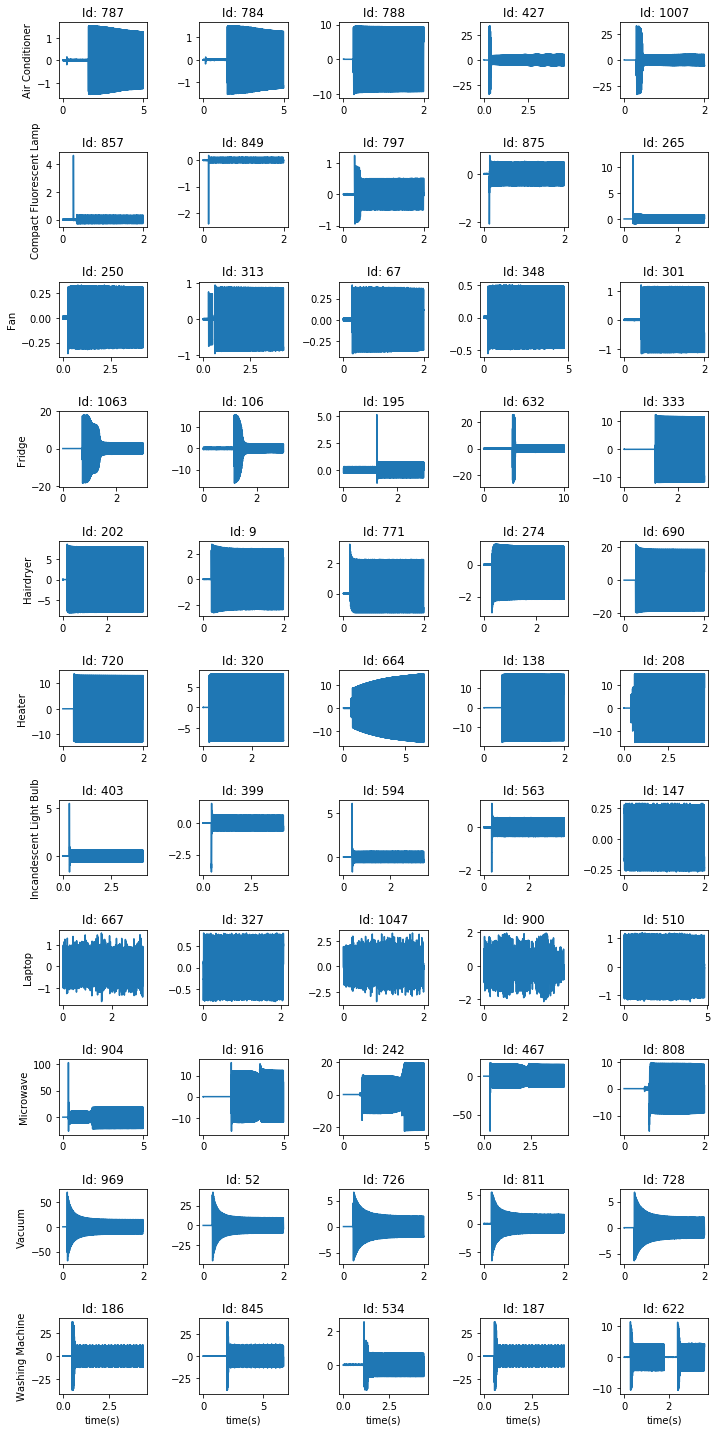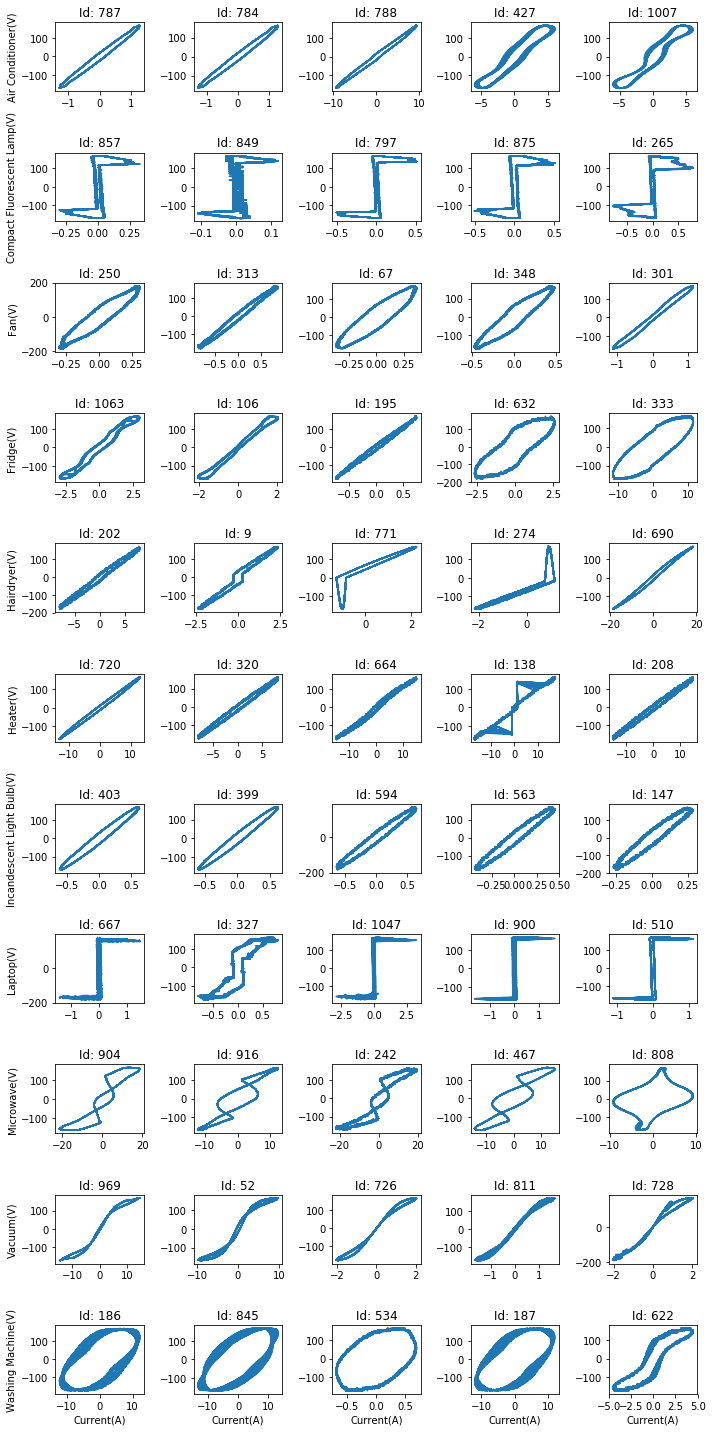Copyright (C) 2015 Jingkun Gao ([email protected])

This file is part of PLAID code repo.

This program is free software: you can redistribute it and/or modify
the Free Software Foundation, either version 3 of the License, or
(at your option) any later version.

This program is distributed in the hope that it will be useful,
but WITHOUT ANY WARRANTY; without even the implied warranty of
MERCHANTABILITY or FITNESS FOR A PARTICULAR PURPOSE.  See the
GNU General Public License for more details.

You should have received a copy of the GNU General Public License
along with this program.  If not, see <http://www.gnu.org/licenses/>

# Initial Expoloreation of PLAID dataset¶

In this IPython Notebook, I will show how to parse the data from CSV files and get the corresponding meta data from JSON files. Meanwhile, I will plot a few appliance intances from each type.

Please make sure Python3 and other necessary packages (using **pip3 -r requirements.txt**) are installed,

In :
# !pip3 install -r requiements.txt


We start from looking at the files under the path of the Dataset. The foler CSV contains csv files represnting all the appliance data, where each file have two columns, the first for current and the second for voltage. The name of the file is an integer starting from 1 reprsenting the instance id. All the associated meta data information can be found in meta1.json.

meta1.json contains the meta data collected in July, 2013, where only the appliance type information is recoreded. For some appliances with multiple states, the transition of different states are also recorded.

In :
Data_path = 'data/'
%ls \$Data_path

CSV/        meta1.json*


The initial thought is to load all csv files into the memory. However, we noticed that all csv files are too huge to be loaded into the memory, so we wrote a function to read data from the CSV path given a list of instance ids (which are the file names).

In :
import os
import numpy as np
import matplotlib.pyplot as plt
%matplotlib inline

csv_path = Data_path + 'CSV/'
csv_files = os.listdir(csv_path)

In :
import subprocess

'''read data given a list of ids and CSV paths'''
n = len(ids)
if n == 0:
return {}
else:
data = {}
for (i,ist_id) in enumerate(ids, start=1):
if progress:
if last_offset==0:
data[ist_id] = np.genfromtxt(path+str(ist_id)+'.csv',
delimiter=',',names='current,voltage',dtype=(float,float))
else:
p=subprocess.Popen(['tail','-'+str(int(offset)),path+
str(ist_id)+'.csv'],stdout=subprocess.PIPE)
data[ist_id] = np.genfromtxt(p.stdout,delimiter=',',
names='current,voltage',dtype=(float,float))
return data


Then, we use JSON package to load meta data.

In :
import json

with open(Data_path + 'meta1.json') as data_file:

meta = [meta1]

In :
meta1

Out:
{'id': '1',
'meta': {'appliance': {'brand': '',
'current': '',
'manufacture_year': '',
'model_number': '',
'notes': '',
'voltage': '',
'wattage': ''},
'notes': 'some instances are not well calibrated, meta data are not complete',
'sampling_frequency': '30000Hz'},
'instances': {'length': '2.00s', 'status': 'off-on'},
'location': 'house1',
'type': 'Compact Fluorescent Lamp'}}

We define two functions to parse meta data stored in JSON format.

In :
def clean_meta(ist):
'''remove '' elements in Meta Data '''
clean_ist = ist.copy()
for k,v in ist.items():
#         if
if len(v) == 0:
del clean_ist[k]
return clean_ist

def parse_meta(meta):
'''parse meta data for easy access'''
M = {}
for m in meta:
for app in m:
M[int(app['id'])] = clean_meta(app['meta'])
return M


In :
Meta = parse_meta(meta)


We can then check the associated meta data given instance id (an interger starting from 1). One examples can be seen below.

In :
Meta

Out:
{'appliance': {'brand': '',
'current': '',
'manufacture_year': '',
'model_number': '',
'notes': '',
'voltage': '',
'wattage': ''},
'notes': 'some instances are not well calibrated, meta data are not complete',
'sampling_frequency': '30000Hz'},
'instances': {'length': '2.00s', 'status': 'off-on'},
'location': 'house51',
'type': 'Laptop'}

We extracted type information for all instances by checking the key "type".

In :
# applinace types of all instances
Types = [x['type'] for x in Meta.values()]
# unique appliance types
Unq_type = list(set(Types))
Unq_type.sort()
print(Unq_type)

# houses
Houses = [x['meta']['location'] for x in meta1]
# appliances
Apps = [x['meta']['location']+'-'+x['meta']['type'] for x in meta1]

['Air Conditioner', 'Compact Fluorescent Lamp', 'Fan', 'Fridge', 'Hairdryer', 'Heater', 'Incandescent Light Bulb', 'Laptop', 'Microwave', 'Vacuum', 'Washing Machine']

In :
print('number of total instances: %d' % len(Types))
print('number of unique households: %d' % len(set(Houses)))
print('number of unique appliances: %d' % len(set(Apps)))
print('number of unique appliance types: %d' % len(Unq_type))

number of total instances: 1074
number of unique households: 55
number of unique appliances: 230
number of unique appliance types: 11


## Plot Instances from PLAID¶

In this section, we will explore instances from differnt appliance types.

At first, we would like to see the statistics of each appliances types.

In :
print('%25s\t%8s\t%8s' % ('Appliance Type', '# Appliances', '# Instances'))
print('-'*70)
for t in Unq_type:
app_ids = [i for i in Apps if t in i]
t_ids = [i for i,j in enumerate(Types) if j == t]
print('%25s\t%10d\t%10d' % (t,len(set(app_ids)),len(t_ids)))
print('-'*70)
print('%25s\t%10d\t%10d' % ('Total',len(set(Apps)),len(Types)))

           Appliance Type	# Appliances	# Instances
----------------------------------------------------------------------
Air Conditioner	        14	        66
Compact Fluorescent Lamp	        31	       175
Fan	        23	       115
Fridge	        21	        38
Hairdryer	        32	       156
Heater	         7	        35
Incandescent Light Bulb	        23	       114
Laptop	        34	       172
Microwave	        28	       139
Vacuum	         8	        38
Washing Machine	         9	        26
----------------------------------------------------------------------
Total	       230	      1074


Then, we will randomly draw 5 instances from each type and plot them. Since voltage normally has a standard shape, we will only draw current at first

In :
# get 5 random instances from each type, will take ~ 1min to run
import random

count = 5
num_type = len(Unq_type)
fs = 30000

ids_to_draw = {}
t_data = {}
for (ii,t) in enumerate(Unq_type):
t_ids = [i for i,j in enumerate(Types,start=1) if j == t]
ids_to_draw[t] = random.sample(t_ids, count)

In :
# plot current
fig = plt.figure(figsize=(10,20))
ids_to_draw = {}
for (ii,t) in enumerate(Unq_type):
jj = 0
for (k,v) in t_data[t].items():
plt.subplot(num_type,count,ii*count+jj+1)
plt.plot(np.linspace(1./fs,len(v['current'])/fs,num=len(v['current'])),v['current'])
if ii==num_type-1:
plt.xlabel('time(s)')
if jj==0:
plt.ylabel(t,fontsize=10)
plt.title('Id: '+str(k))
jj += 1
fig.tight_layout()
plt.show()For a better visualization of the dataset to consider voltage, let's look at the V-I trajectory. To make it easier to be seen, we will look at the last 10000 points, which reprsents 20 periods of steady states.

In :
# plot V-I of last 10 steady state periods
fig = plt.figure(figsize=(10,20))
ids_to_draw = {}
for (ii,t) in enumerate(Unq_type):
jj = 0
for (k,v) in t_data[t].items():
plt.subplot(num_type,count,ii*count+jj+1)
plt.plot(v['current'][-10000:],v['voltage'][-10000:])
if ii==num_type-1:
plt.xlabel('Current(A)')
if jj==0:
plt.ylabel(t+ '(V)',fontsize=10)
plt.title('Id: '+str(k))
jj += 1
fig.tight_layout()
plt.show()From the shapes of V-I, we can clearly see the distinct behaviours of appliances of different type. Although, we also noticed that for some appliances of the same type, they show varient patterns.

To confirm the meta data information, we can use

In :
Meta

Out:
{'appliance': {'brand': '',
'current': '',
'manufacture_year': '',
'model_number': '',
'notes': '',
'voltage': '',
'wattage': ''},
'type': 'Washing Machine'}